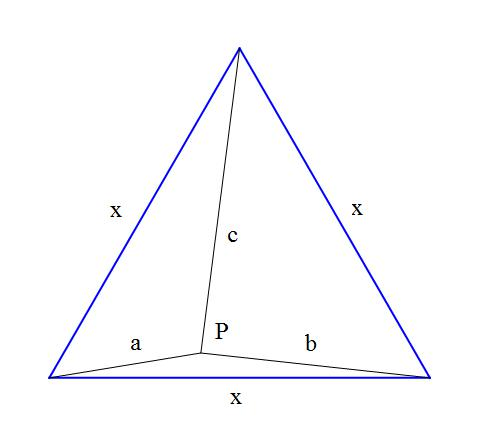# Equilateral Triangle Dissection

Geometry Level 5

An equilateral trianglehas an interior point $P$ from which the distances to the $3$ vertices are distinct integers $a\neq b\neq c\neq a$ that satisfy the condition

$2\Delta xxx=\Delta aaa+\Delta bbb+\Delta ccc+3\Delta abc$

where the notation $\Delta abc$ shall denote the non-zero area of a triangle with sides $a, b, c$, and $x$ is the side of the equilateral triangle.

Find the minimum value $a+b+c$ can have.

×